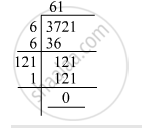Advertisement Remove all ads

# Find the Square Root Of: 75 46 49 - Mathematics

Find the square root of:

$75\frac{46}{49}$

Advertisement Remove all ads

#### Solution

We know:

$\sqrt{75\frac{46}{49}} = \sqrt{\frac{3721}{49}} = \frac{\sqrt{3721}}{\sqrt{49}}$

Now, let us compute the square roots of the numerator and the denominator separately.$\sqrt{49} = 7$
$\therefore \sqrt{75\frac{46}{49}} = \frac{61}{7}$

Is there an error in this question or solution?
Advertisement Remove all ads

#### APPEARS IN

RD Sharma Class 8 Maths
Chapter 3 Squares and Square Roots
Exercise 3.6 | Q 1.08 | Page 48
Advertisement Remove all ads

#### Video TutorialsVIEW ALL 

Advertisement Remove all ads
Share
Notifications

View all notifications

Forgot password?
Course